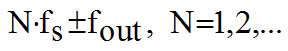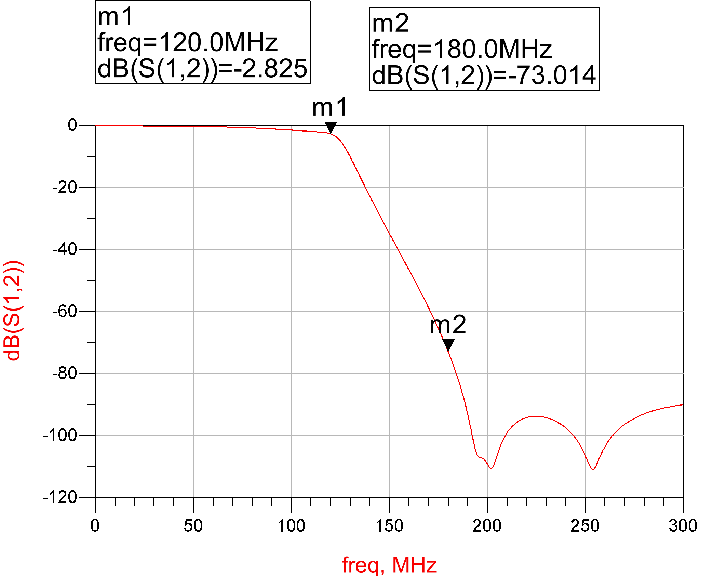1 Preface
In the data sheet for Siglent’s SDG2000X arbitrary waveform generator series, the sampling rate specification (1.2GSa/s) is followed by a note of “4X Interpolation”.

 Sampling Rate 1.2 GSa/s (4X Interpolation) Vertical Resolution 16 bit

This be confusing to some customers, even some experienced users of function generators. This white paper is intended to explain this specification and to discuss the advantages of the Interpolated DDS technique compared to a traditional DDS based function generator.

The figure below shows the basic design of the traditional DDS technique,in which digital waveform data is output in response toa reference clock for the DDS circuitry, then converted by the D/A converter using the same clock. That is to say, in a traditional DDSstructure the clock for the DDS and for the D/A converter run at the same frequency.

A reconstruction filter following the D/A converter helps to smooth the steps from the D/A’s output in order to produce a smoother, more “analog” signal.

3 Introduction to Interpolated DDS
The figure below shows the structure of the Interpolated DDS. An interpolator is inserted between the DDS circuitry and D/A converter. In this structure, digital waveform data is output in response to the reference clock of the DDS circuitry, interpolated by “I” (in SDG2000X I = 4), and then converted by the D/A, with a sampling clock“I” times the frequency of the reference clock.After the D/A convertor, a reconstruction filter is also applied.

Siglent’sSDG2000X applies this technique with the interpolation factor I = 4. The reference clock of the DDS circuitry on the SDG2000X is 300MHz.Using the interpolation circuitry, the sampling clock of the D/A convertor is increased to 1.2GHz. This is the explanation behind 1.2 GSa/s specification found in the data sheet – 1.2GSa/s followed by a “4X interpolation” note.

This explains the mechanics behind the interpolated sampling technique.However, what is the technical reasoning behind Siglent’s innovative sampling technique?

4 Why Interpolation?
4.1 To Break the Bandwidth Limit
First, let’s compare the waveform in the time domain using the two different sample rates.
Looking at the output waveform of the D/A, the 1.2GSa/s sample rate results in smaller steps (higher resolution) than with the 300MSa/s sampling clock (Fig 3).

The reader may ask, “If both waveforms are to be passed through a restructuring filter in order to smooth the waveform steps, then the sample rate should not matter.” The fact is, the step size does affect the final smoothed waveform.

Now let’s look at this difference in the frequency domain in order to get a clearer understanding.

The figure below shows a spectrum example in which an 80MHz sine wave is output by a D/A with 300MSa/s. Output spectrum of the D/A includes the fundamental, and its image frequencies.Amplitudes of all the spectrum components comply with the sin(x)/x envelope. Usage of the reconstruction filter is to filter all of the image frequencies which are located outside the Nyquist bandwidth.

An ideal reconstruction filter should retain all the signals located in the Nyquist bandwidth, and filter out the ones outside it. In other words, its frequency response should be coincident with the Nyquist bandwidth, as the shadow part in Figure 4.In this case, the maximum frequency can reach the Nyquist Limit (e.g. 1/2 sampling clock frequency).

As we know, in engineering the ideal filter with “brick wall” response doesn’t exist. In the real world, filters actually have some degree of roll off.

Figure 4 shows the spectrum of the output from a 300 MSa/s A/D converter with an output frequency of 80 MHz. The nearest image occurs at 220 MHz so the maximum roll off would occur at 140 MHz.

But for a 150MHz signal, the nearest image is 300 – 150 = 150MHz, the space remaining for roll off is zero, which is not realizable. Generally, the limit bandwidth of a reconstruction filter is 40% of the sampling clock. That is to say, for a 300MSa/s D/A, the maximum available output frequency is 120MHz.

The figure below shows 2 reconstruction filter designs with roll offs from 120MHz to 180MHz.Both designs are 9-order elliptic filters.The difference is the design on the left uses ideal components, and the one on the right uses real-world components, with parasitic parameters. As we can see, a real filter has an extra ~3dB attenuation on the corner frequency (i.e. 120MHz), and its attenuation performance on the stop band cutoff frequency (i.e. 180MHz) degrades.In addition, the sin(x)/x envelope of the D/A’s response itself will add attenuation to the signal. At 40% of the sampling clock (e.g. 120MHz in Figure 4), the attenuation caused by the D/A is about 2.4dB. An inverse sin(x)/x filter is generally necessary to compensate this attenuation.

If the sampling rate of D/A is 1.2GSa/s, for a 80MHz signal, the nearest image is 1.12GHz, so the maximum roll off of reconstruction filter could be 1.04GHz, as shown in Figure 6. Design of the reconstruction filter is greatly simplified.